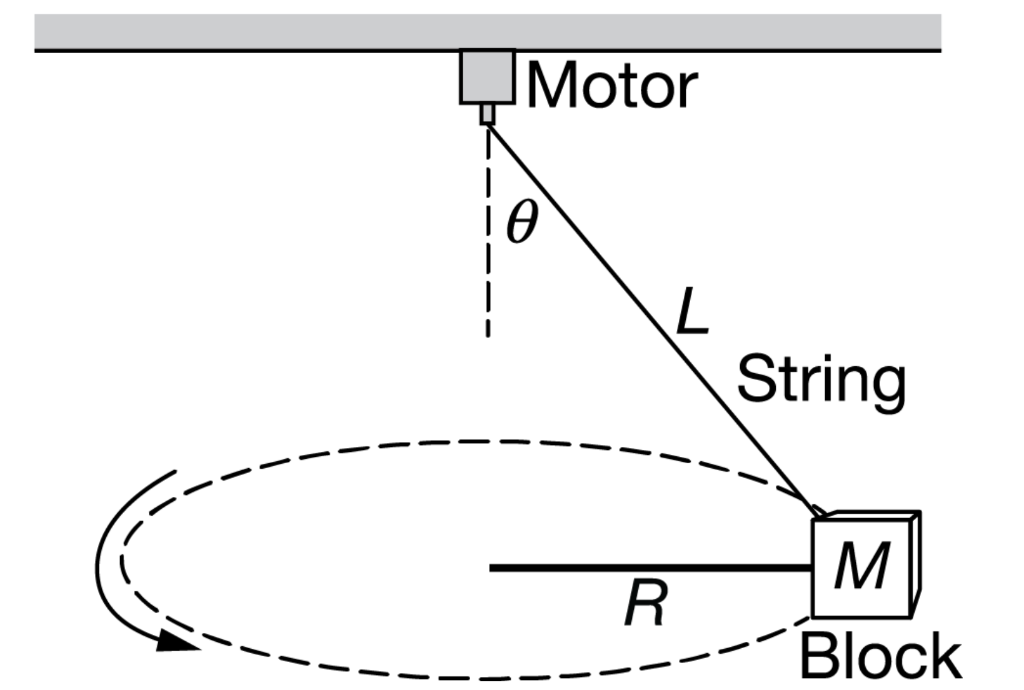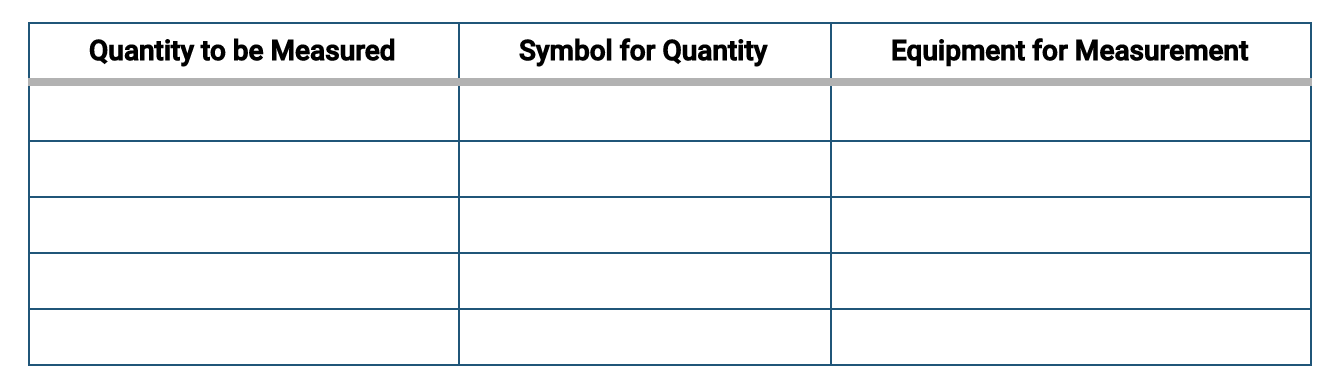# Question Solved1 AnswerIn the apparatus shown above, one end of a string of length L is attached to a block of mass M and the other end is connected to the axle of a motor that rotates, causing the block to move in a circle of radius R at a constant speed vT such that the string makes an angle θ with the vertical. A student wants to use the apparatus to make measurements and create a graph that can be used to estimate the acceleration g due to gravity at the surface of Earth. The student can adjust the motor to achieve different tangential speeds of the block. (b) Using the experimental apparatus shown above and the available equipment, design an experimental procedure the student could use to estimate the acceleration g due to gravity at the surface of Earth. i. In the table below, list the quantities and associated symbols that would be measured in the experiment and the equipment used to measure them. Also list the equipment that would be used to measure each quantity. You do not need to fill in every row. If you need additional rows, you may add them to the space just below the table. ii. Describe an experimental procedure to estimate the acceleration g due to gravity at the surface of Earth using the apparatus. Give enough detail so that another student could replicate the experiment. As needed, include a diagram of the experimental setup. Assume equipment usually found in a school physics laboratory is available. (c) The student wants to analyze data collected from the experiment by creating a linear graph that can be used to estimate the acceleration g due to gravity at the surface of Earth. i. State what quantity should be plotted on the horizontal axis and what quantity should be plotted on the vertical axis to produce the linear graph. Horizontal axis: ____________ Vertical axis: _____________ ii. Indicate which feature of the graph could be used to determine g. Motor String M -- Block - - - - - - - - Quantity to be Measured Symbol for Quantity Equipment for MeasurementIn the apparatus shown above, one end of a string of length L is attached to a block of mass M and the other end is connected to the axle of a motor that rotates, causing the block to move in a circle of radius R at a constant speed vT such that the string makes an angle θ with the vertical. A student wants to use the apparatus to make measurements and create a graph that can be used to estimate the acceleration g due to gravity at the surface of Earth. The student can adjust the motor to achieve different tangential speeds of the block.

(b) Using the experimental apparatus shown above and the available equipment, design an experimental procedure the student could use to estimate the acceleration g due to gravity at the surface of Earth.

i. In the table below, list the quantities and associated symbols that would be measured in the experiment and the equipment used to measure them. Also list the equipment that would be used to measure each quantity. You do not need to fill in every row. If you need additional rows, you may add them to the space just below the table.ii. Describe an experimental procedure to estimate the acceleration g due to gravity at the surface of Earth using the apparatus. Give enough detail so that another student could replicate the experiment. As needed, include a diagram of the experimental setup. Assume equipment usually found in a school physics laboratory is available.

(c) The student wants to analyze data collected from the experiment by creating a linear graph that can be used to estimate the acceleration g due to gravity at the surface of Earth.

i. State what quantity should be plotted on the horizontal axis and what quantity should be plotted on the vertical axis to produce the linear graph.

Horizontal axis: ____________

Vertical axis: _____________

ii. Indicate which feature of the graph could be used to determine g.

Transcribed Image Text: Motor String M -- Block - - - - - - - - Quantity to be Measured Symbol for Quantity Equipment for Measurement
More
Transcribed Image Text: Motor String M -- Block - - - - - - - - Quantity to be Measured Symbol for Quantity Equipment for Measurement